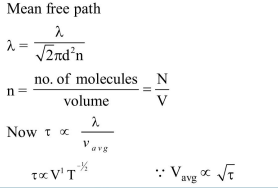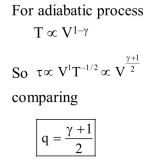# Consider an ideal gas confined`
Question:

Consider an ideal gas confined in an isolated closed chamber. As the gas undergoes an adiabatic expansion, the average time of collision between molecules increases as $\mathrm{Vq}$, where $\mathrm{V}$ is the volume of the gas. The value of $q$ is :- $\left(\gamma=\frac{C_{p}}{C_{v}}\right)$

1. $\frac{\gamma+1}{2}$

2. $\frac{\gamma-1}{2}$

3. $\frac{3 \gamma+5}{6}$

4. $\frac{3 \gamma-5}{6}$

Correct Option: 1

Solution: